# Bar Charts

## Data

Most of the following examples analyze data in the Employee data set, included with lessR. To read an internal lessR data set, just pass the name of the data set to the lessR function Read(). Read the Employee data into the data frame d. See the Read and Write vignette for more details.

d <- Read("Employee")
##
## >>> Suggestions
##
## Data Types
## ------------------------------------------------------------
## character: Non-numeric data values
## integer: Numeric data values, integers only
## double: Numeric data values with decimal digits
## ------------------------------------------------------------
##
##     Variable                  Missing  Unique
##         Name     Type  Values  Values  Values   First and last values
## ------------------------------------------------------------------------------------------
##  1     Years   integer     36       1      16   7  NA  15 ... 1  2  10
##  2    Gender character     37       0       2   M  M  M ... F  F  M
##  3      Dept character     36       1       5   ADMN  SALE  SALE ... MKTG  SALE  FINC
##  4    Salary    double     37       0      37   53788.26  94494.58 ... 56508.32  57562.36
##  5    JobSat character     35       2       3   med  low  low ... high  low  high
##  6      Plan   integer     37       0       3   1  1  3 ... 2  2  1
##  7       Pre   integer     37       0      27   82  62  96 ... 83  59  80
##  8      Post   integer     37       0      22   92  74  97 ... 90  71  87
## ------------------------------------------------------------------------------------------

As an option, also read the table of variable labels. Create the table formatted as two columns. The first column is the variable name and the second column is the corresponding variable label. Not all variables need be entered into the table. The table can be a csv file or an Excel file.

Currently, read the label file into the l data frame. The labels will be displayed on both the text and visualization output. Each displayed label is the variable name juxtaposed with the corresponding label.

l <- rd("Employee_lbl")
##
## >>> Suggestions
##
## Data Types
## ------------------------------------------------------------
## character: Non-numeric data values
## ------------------------------------------------------------
##
##     Variable                  Missing  Unique
##         Name     Type  Values  Values  Values   First and last values
## ------------------------------------------------------------------------------------------
##  1     label character      8       0       8   Time of Company Employment ... Test score on legal issues after instruction
## ------------------------------------------------------------------------------------------

## Bar Chart of One Variable

One of the most frequently encountered visualizations is the bar chart, created for the values of a categorical variable that are each associated with a corresponding value of a numerical variable.

Bar chart: Plot a bar for each level of a categorical variable with its height scaled according to the value of an associated numerical variable.

A call to a function to create a bar chart contains the name of the categorical variable with the categories to be plotted. With the BarChart() function, that variable name is the first argument passed to the function. In this example, the only argument passed to the function is the variable name as the data frame is named d, the lessR default value. Or, specify the data frame that contains the variable(s) of interest with the data parameter.

The following illustrates the call to BarChart() with a categorical variable named $$x$$.When only a single categorical variable is passed to BarChart(), the numerical value associated with each bar is the corresponding count of the number of occurrences, automatically computed.

### Default Bar Chart

Consider the categorical variable Dept in the Employee data table. Use BarChart() to tabulate and display the visualization of the number of employees in each department, here relying upon the default data frame (table) named d. Otherwise add the data= option if the data frame has another name.

BarChart(Dept)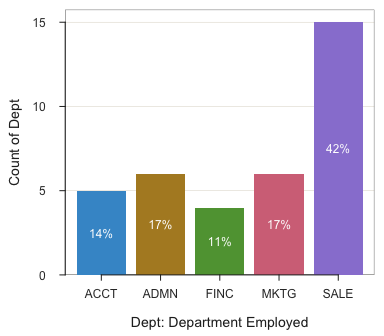Bar chart of tablulated counts of employees in each department.

## >>> Suggestions
## BarChart(Dept, horiz=TRUE)  # horizontal bar chart
## BarChart(Dept, fill="reds")  # red bars of varying lightness
## PieChart(Dept)  # doughnut (ring) chart
## Plot(Dept)  # bubble plot
## Plot(Dept, stat="count")  # lollipop plot
##
##
## --- Dept: Department Employed ---
##
##
## Missing Values of Dept: 1
##
##
##                 ACCT   ADMN   FINC   MKTG   SALE    Total
## Frequencies:       5      6      4      6     15       36
## Proportions:   0.139  0.167  0.111  0.167  0.417    1.000
##
##
## Chi-squared test of null hypothesis of equal probabilities
##   Chisq = 10.944, df = 4, p-value = 0.027

The default color theme, "colors", fills the bars in the bar chart with with different hues. See more explanation of this and related color palettes more in the vignette Customize.

The BarChart() function also labels each bar with the associated numerical value. The function also provides the corresponding frequency distribution, the table that lists the count of each category, from which the bar chart is constructed.

We do not need to see this output to the R console repeated again for different bar charts of the same data, so turn off for now with the parameter quiet set to TRUE. Can set this option for each call to BarChart(), or can set as the default for subsequent analyses with the style() function.

style(quiet=TRUE)

### Custom Colors

Specify a single fill color with the fill parameter, the edge color of the bars with color. Set transparency level with trans. Against a lighter background, display the value for each bar with a darker color using the values_color parameter. To specify a color, use color names, specify a color with either its rgb() or hcl() color space coordinates, or use the lessR custom color palette function getColors().

BarChart(Dept, fill="darkred", color="black", trans=.8, values_color="black")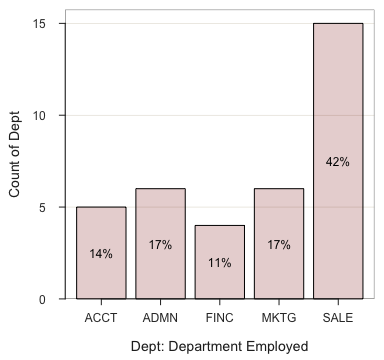Use the theme parameter to change the entire color theme: “colors”, “lightbronze”, “dodgerblue”, “darkred”, “gray”, “gold”, “darkgreen”, “blue”, “red”, “rose”, “green”, “purple”, “sienna”, “brown”, “orange”, “white”, and “light”. In this example, changing the full theme accomplishes th same as changing the fill color. Turn off the displayed value on each bar with the parameter values set to off. Specify a horizontal bar chart with base R parameter horiz.

BarChart(Dept, theme="gray", values="off", horiz=TRUE)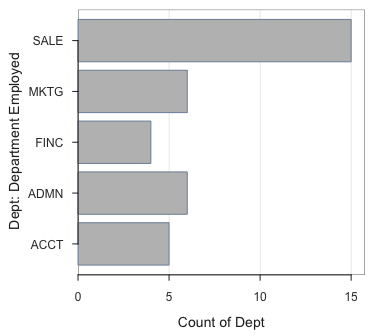Or, can use style() to change the theme for subsequent visualizations as well. See the Customize vignette.

Dept is not an ordinal variable (i.e., with ordered values set by the base R factor() function). Ordinal varaibles plot by default with a range of the same hue from light to dark. To illustrate, can choose many different sequential palettes from getColors(): “reds”, “rusts”, “browns”, “olives”, “greens”, “emeralds”, “turquoises”, “aquas”, “blues”, “purples”, “violets”, “magentas”, and “grays”.

BarChart(Dept, fill="reds")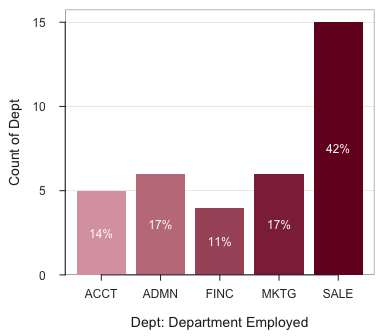### Rotate Labels

Rotate and offset the axis labels with rotate_x and offset parameters. Do a descending sort of the categories by frequencies with the sort parameter.

BarChart(Dept, rotate_x=45, offset=1, sort="-")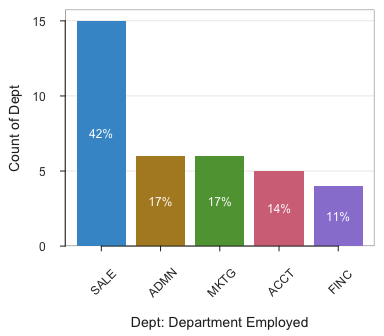Instead of arbitrarily setting the value of the interior color of the bars with the fill parameter, map the value of the tabulated count to the bar fill. With mapping, the color of the bars depends upon the bar height. The higher the bar, the darker the color. Specify (count) as the fill color to map the values of the numerical variable to the fill color.

BarChart(Dept, fill=(count))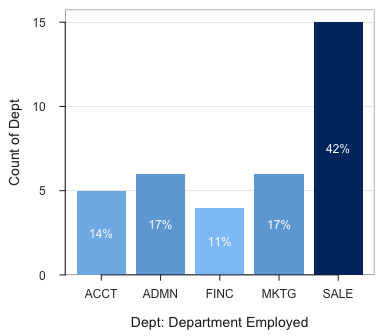### Specify the Numerical Variable

One possibility begins with the values of the $$x$$ and $$y$$ variables, such as in a table, and then create the bar chart directly from this summary table. To do so, enter the paired data values into a data file such as with Excel, and then read into R with Read(). When calling BarChart(), specify the categorical $$x$$ variable and then the numerical $$y$$ variable.

When the numeric variable is specified, the data are a summary (pivot) table, with one row for each level of the categorical variable plotted. For example, suppose a summary table contains the departments and the mean salary for each department. Obtain the summary table with the lessR pivot() function (which has its own vignette). For the data frame d, calculate the mean of numerical variable Salary across levels of the categorical variable Dept.

a <- pivot(d, mean, Salary, Dept)
a
##   Dept n_Salary na_Salary Salary_mn
## 1 ACCT        5         0  61792.78
## 2 ADMN        6         0  81277.12
## 3 FINC        4         0  69010.68
## 4 MKTG        6         0  70257.13
## 5 SALE       15         0  78830.07

The general syntax follows for processing this form of the data follows.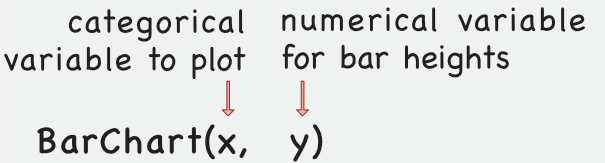The bar chart follows, with the aggregated data stored in the data frame named a, so explicitly identify with the data parameter. The computed mean of Salary variable in the a data frame from the previous call to pivot() is named Salary_mn by default.

BarChart(Dept, Salary_mn, data=a)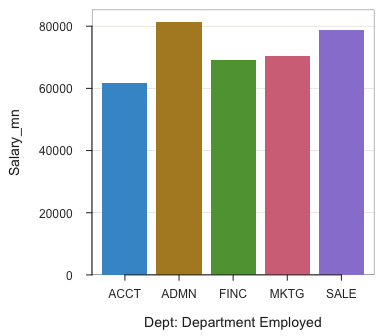### Statistical Transformation of $$y$$

Can also do a statistical transformation of the numerical value of $$y$$ with the stat parameter. Possible values of stat: "sum", "mean", "sd", "dev", "min", "median", and "max". The "dev" value displays the mean deviations to further facilitate a comparison among levels.

Here the $$x$$-variable is Dept, and $$y$$-variable is Salary. Display bars for values of dev <= 0 in a different color than values above with the fill_split parameter set at 0. Do an ascending sort with the sort parameter set at "+".

BarChart(Dept, Salary, stat="dev", sort="+", fill_split=0)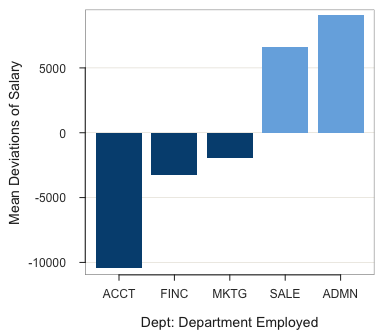Compare this visualization of the mean deviations with the previous visualization of the means for each Dept.

### Annotation

Annotate a plot with the add parameter. To add a rectangle use the "rect" value of add. Here set the rectangle around the message centered at <3,10>. To specify a rectangle requires two corners of the rectangle, <x1,y1> and <x2,y2>. To specify text requires just a single coordinate, <x1,y1>. Because with the add parameter, the message follows the specification of "rect", the coordinates of the text message follow the coordinates for the rectangle.

First lighten the fill color of the annotation with the add_fill parameter for the style() function.

style(add_fill="aliceblue")
x1=c(1.75,3), y1=c(11, 10), x2=4.25, y2=9)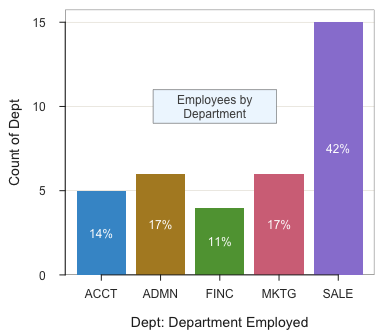## Pie Chart

An alternative to the bar chart for a single categorical variable is the pie chart.

Pie Chart: Relate each level of a categorical variable to the area of a circle (pie) scaled according to the value of an associated numerical variable.

Here the presented version of a pie chart is the doughnut or ring chart.

PieChart(Dept)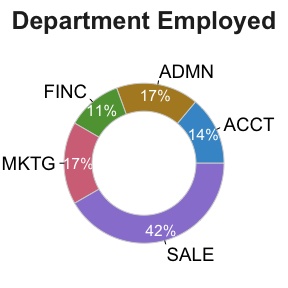The doughnut or ring chart appears easier to read than a standard bar chart. But the lessR function PieChart() also can create the “old-fashioned” pie chart by setting the value of hole to 0. We have seen the summary statistics several times now, so turn off the output to the R console here with the quiet parameter.

PieChart(Dept, hole=0, quiet=TRUE)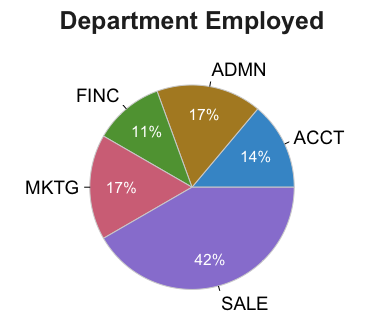Standard pie chart of variable Dept in the d data frame.

Set the size of the hole in the doughnut or ring chart with the parameter hole, which specifies the proportion of the pie occupied by the hole. The default hole size is 0.65. Set that value to 0 to close the hole.

## Bar Chart of Two Variables

### Standard Bar Charts

Specify the second categorical variable with the by parameter. Specify the by parameter by name. The general syntax follows.The example plots Dept with the percentage of Gender divided in each bar.

BarChart(Dept, by=Gender)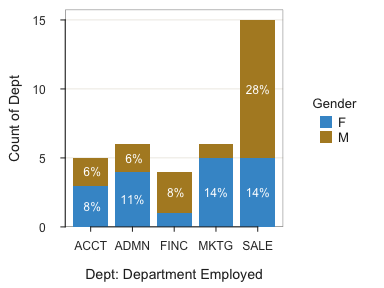Specify two custom fill colors for Gender.

BarChart(Dept, by=Gender, fill=c("deepskyblue", "black"))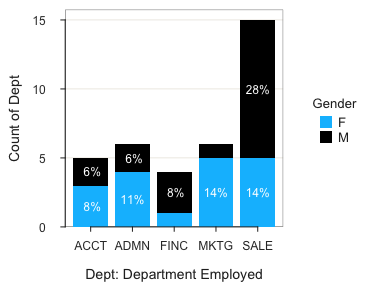The stacked version is default, but the values of the second categorical variable can also be represented with bars, more helpful to compare the values with each other. Here put the legend on the top with the values_position parameter set to "out".

BarChart(Dept, by=Gender, beside=TRUE, values_position="out")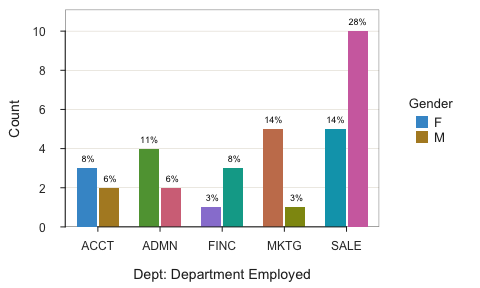Or, display the bars horizontally with the horiz parameter set to TRUE.

BarChart(Gender, by=Dept, horiz=TRUE)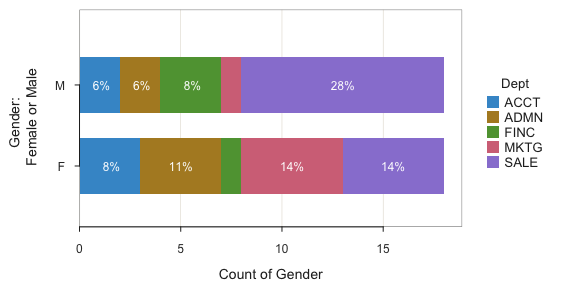### Trellis Plots

Can also do a Trellis chart with the by1 parameter.

BarChart(Dept, by1=Gender)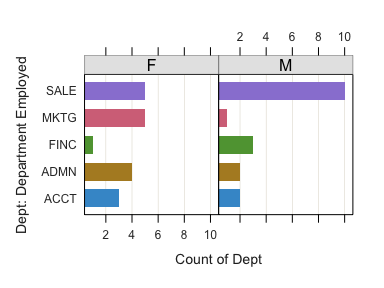Or, stack the charts vertically by specifying one column with the n_col parameter.

BarChart(Dept, by1=Gender, n_col=1)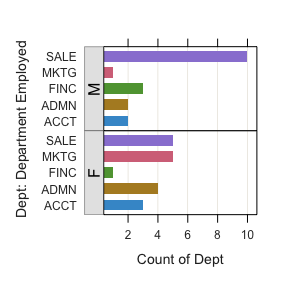### 100% Stacked Bar Chart

Obtain the 100% stacked version with the stack100 parameter. This visualization is most useful for comparing levels of the by variable across levels of the x variable, here Dept, when the frequencies in each level of the x variable differ. The percentages across categories are compared instead of the counts. The percentage for each column, then, sums to 100%.

BarChart(Dept, by=Gender, stack100=TRUE)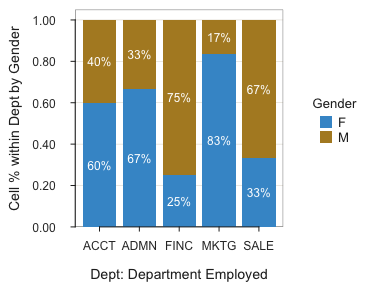### Long Value Labels

Long value labels on the horizontal axis are also addressed by moving to a new line whenever a space is encountered in the label. Here read responses to the Mach IV Machiavellianism scale where each item is scored from 0 to 5.

d <- rd("Mach4", quiet=TRUE)

Also read variable labels into the l data frame, which are then used to automatically label the output, both the visualization and text output to the console.

l <- rd("Mach4_lbl", quiet=TRUE)

Convert the specified four Mach items to factors with the lessR function factors(). A response of 0 is a Strongly Disagree, etc.

LikertCats <- c("Strongly Disagree", "Disagree", "Slightly Disagree",
"Slightly Agree", "Agree", "Strongly Agree")
d <- factors(c(m06,m07,m09,m10), levels=0:5, labels=LikertCats, ordered=TRUE)

Because the factors are defined as ordered in the factors() function, the colors are plotted in a sequential scale, from light to dark. Because output to the console has been turned off in general, turn back on just for this analysis because of new data.

BarChart(m06, by=m07, quiet=FALSE)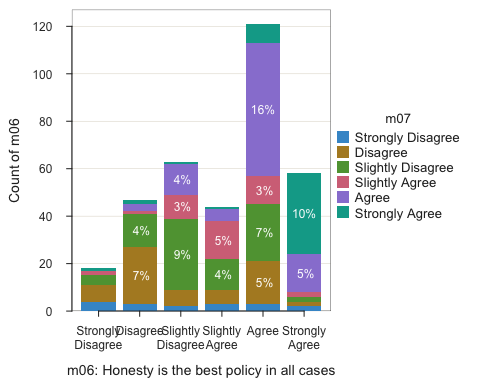## >>> Suggestions
## Plot(m06, m07)  # bubble plot
## BarChart(m06, by=m07, horiz=TRUE)  # horizontal bar chart
## BarChart(m06, fill="steelblue")  # steelblue bars
##
##
## m06: Honesty is the best policy in all cases
##   - by levels of -
## m07: There is no excuse for lying to someone else
##
## Joint and Marginal Frequencies
## ------------------------------
##
##                    m06
## m07                 Strongly Disagree Disagree Slightly Disagree Slightly Agree Agree Strongly Agree Sum
##   Strongly Disagree                 4        3                 2              3     3              2  17
##   Disagree                          7       24                 7              6    18              2  64
##   Slightly Disagree                 4       14                30             13    24              2  87
##   Slightly Agree                    2        1                10             16    12              2  43
##   Agree                             0        3                13              5    56             16  93
##   Strongly Agree                    1        2                 1              1     8             34  47
##   Sum                              18       47                63             44   121             58 351
##
##
## Cramer's V: 0.380
##
## Chi-square Test:  Chisq = 253.103, df = 25, p-value = 0.000
## >>> Low cell expected frequencies, chi-squared approximation may not be accurate

## Full Manual

Use the base R help() function to view the full manual for BarChart(). Simply enter a question mark followed by the name of the function.

?BarChart

## More

More on Bar Charts and other visualizations from lessR and other packages such as ggplot2 at:

Gerbing, D., R Visualizations: Derive Meaning from Data, CRC Press, May, 2020, ISBN 978-1138599635.